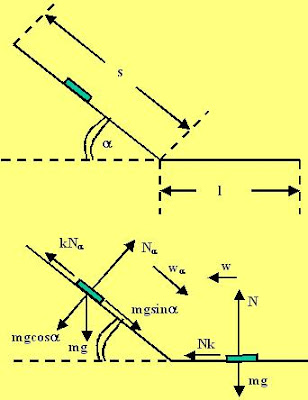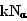Thursday, February 14, 2008

Irodov Problem 1.122Suppose that the disc started its journey down the slope from a point s units from the horizontal as shown in the figure. We shall consider the disc's motion on the slope and the horizontal separately.

Motion down the slope: There are three forces acting on the disc along the slope, i) force of gravity mg, ii) the normal reactionand iii) the force of friction opposing its motion. Let the acceleration of the mass down the slope be. Then as shown in the diagram we have,Motion along the horizontal plane:
Along the horizontal plane force of friction decelerates at a rate w the disc until it stops. The force equations are given below,The disc started with 0 velocity, achieved maximum velocity at the bend after traveling a distance of s and then stopped after traveling a distance of l. Suppose that the maximum
velocity it achieved was v, then,The work done by friction along the slope is(the negative sign because the direction of motion is in the opposite direction of the motion). The work done by the force of friction on the horizontal plane is -kmgl. So the total work done by the force of friction is given by,